﻿ Quantization of Space-Time Explains Matter and Its AspectsInternational Journal of Theoretical and Mathematical Physics

p-ISSN: 2167-6844    e-ISSN: 2167-6852

2020;  10(4): 83-90

doi:10.5923/j.ijtmp.20201004.03

Received: Dec. 19, 2019; Accepted: Sep. 9, 2020; Published: Sep. 26, 2020### Quantization of Space-Time Explains Matter and Its Aspects

George Gerhab

Hellertown, USA

Correspondence to: George Gerhab, Hellertown, USA.
 Email: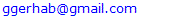Abstract

In this paper, I present a theory that by quantizing space-time in such a way that I can calculate all of the constants of physics and derive and explain every equation therein. The magnetic field appears when the electric field seems to travel faster than c. In the same way an “anti-gravity” perpendicular to the gravity field appears, totally dependent on relative velocities, since the electric and gravity fields are made of the same micro-lines and are different aspects of the same force. This gravitational perpendicular force can be repellent, when masses travel in the same direction (massive distant galaxies travelling at near light speed would repel greatly and enlarge the universe) and it can attractive if the masses are travelling in the same direction (opposite arms of a spiral galaxy) in this paper, the electric and gravitational forces are shown to be unified. The recent detection of gravity waves is evidence for this, since a perpendicular gravity field would be needed to make a wave.

Keywords: Explanation and unification of the electric and magnetic fields, Quantized space-time, Proposed perpendicular gravity field, A theory of one particle and one force

Cite this paper: George Gerhab, Quantization of Space-Time Explains Matter and Its Aspects, International Journal of Theoretical and Mathematical Physics, Vol. 10 No. 4, 2020, pp. 83-90. doi: 10.5923/j.ijtmp.20201004.03.

### 1. Introduction

I propose that the magnetic field appears when the electric field seems to travel faster than c. In the same way I propose an “anti-gravity” perpendicular field appears, totally depended on relative velocities, since the electric and gravitational forces are as I propose in my theory made of the same micro field lines.
The “gravitational” perpendicular force can be repellant, when masses travel in the same direction, (massive distant galaxies, new current mass just being released) and it can be attractive (opposite spiral arms moving in opposite directions, combined with a super-massive black hole).
The recent detection of gravity waves is clear evidence for all of this since these waves need a perpendicular field, like electro-magnetic waves. Units are man-made and involve much more than we can imagine.
The object of this paper is to quantize space-time and show that it can explain the electric and gravitational fields and unify them.

### 2. Quantization of Space and Time

The electron is, by far, the most important particle known. This tiny electric particle is responsible for every bio-chemical reaction and structure in our body as well as every chemical reaction that happens anywhere and it is also the tiny electric messenger for our computers and smartphones.

#### 2.1. Quantization of Space-Time

The electron has been known for over 100 years and is the most studied particle there is and, yet, no one knows what it is.
Perhaps, the reason for not knowing is that both scientists and people consider space and time to be continuous. Everyone knows intuitively what space and time are. Space is the place where objects move, and time is how long it takes the objects to move. Since there are no jumps in time or space, they are considered continuous.
However, the electron cannot exist in a continuous space –time. Scientists have to renormalize (ie, fudge) their equations to account for the electron’s apparently infinite charge and mass by infinitely screening them with virtual pairs of particles. This should say something besides, “?”.
The electron can only exist in a universe where both space and time are quantized.
My idea for Quantization of space means that no two points can get closer than a certain quantized distance, call this distance x0. All distances have to be whole numbers of this distance. Quantization of time means that time progresses in steps of a certain amount of time, call this amount of time t0, rather than proceeding continuously as we believe. All time amounts must be whole numbers of the time t0.

#### 2.2. The Singleton Point and the Explanation Of Matter

I believe that space-time may consist of an infinite ocean of randomly appearing and disappearing pairs of points. One point of each pair jumps backward in time while the other point jumps forward in time. They blink back to zero in time and disappear in t0 amount of time, thus preserving time quantization. The two points are exactly x0 distance apart which preserves space quantization. A point is a singularity which has no dimensions or structure to it. Points, I believe, are the only thing that can exist in the universe, because, if anything else did, with some type of structure, the immediate question would be, “What is it made of?”. This question cannot be answered.
These virtual pairs of points build up space-time. The points are not in space-time, they are space-time. The points in these virtual pairs must always be exactly x0 distance apart. Any other distance would disobey the very structure of space-time itself.
Two points of quantized space-time must share their time. That is, if one point’s time jumps back to zero, another point’s time (x0 away) must jump forward to zero, in the opposite direction, then the points disappear. Virtual space-time points disappear when two points share time in an integral step, in opposite directions (entanglement?). The integrity of the elementary unit of time cannot be violated. It is exactly the same everywhere, no matter what reference frame.
This sharing of time could be the mysterious effect of entanglement and, since the two points sharing time do not have to be the two virtual points that occurred together, entanglement would be a universal property of quantized space-time which could connect all of space-time throughout infinity.
The space quantization is created by the elementary time unit by the equation x0 = ct0 where c is a space-time constant. Since the integrity of space-time cannot be violated, c is a universal constant. In natural units, c = 1, and time and space are one and the same thing. With space quantization, only equilateral triangles are possible, and the myriad of virtual space-time points creates the 3-D effect we are familiar with but any point in a 4th dimensional space would not be possible cause it would have to violate the integrity of quantized space-time.

### 3. The Singleton Point

Now consider a singleton point, which has time but no paired point to share its time within order to disappear. It cannot move anywhere and would appear stuck. But with the vast amounts of virtual pairs constantly appearing and disappearing, one pair will certainly come close enough in order that the singleton point can share its time with one of the points of the virtual pair and disappear. The other point of the virtual pair becomes a new singleton point.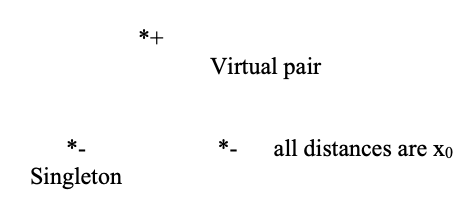Figure 1. Singleton point disappears with + virtual point leaving the – virtual point to become the singleton point

#### 3.1. My Proposal on How Singleton Points Create Matter

The negative time singleton point shares its time with the positive point of the virtual pair. These two points disappear leaving the negative time point as the new singleton point. In this way, the singleton point zips around, at random, at the speed of light and becomes what we call matter. The equation for the random walk problem and Schrödinger’s equation are identical except for the ih’s.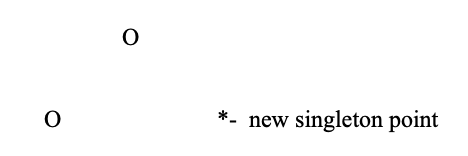Figure 2

#### 3.2. Singleton Point has Moved

The singleton point has now moved a distance of x0 in a time t0 at the speed of light. Such singleton points move over 1044 times every second.
The only other way that this singleton can move is seen in figure 3:Figure 3. Singleton point ready to interact with quantized matter

#### 3.3. Single Point Ready to Interact with Quantized Space-Time

Since all points must be x0 apart, they can only form equilateral triangles, and the only angle that can exist is 60°. When the singleton point shares time with the + virtual point and disappears, we have figure 4:Figure 4. The new singleton point has moved at the speed of light from the old single. In this way a singleton point moves around randomly at the speed of light creating matter
The new singleton point has moved at an angle of 60°, the only angle, besides zero, that the singleton point can travel. Since cos 60° = ½, this is the reason that spin is 1/2.
The singleton point travels randomly at the speed of light within a small volume, since the virtual pairs randomly appear close to the singleton point. According to the random walk problem, this small volume expands (the differential equation for the random walk problem is nearly the same as Schrödinger’ equation).
Since the new singleton point has suddenly appeared, it tries to share time with the virtual pair directly in front of it but it cannot since the new singleton would have travelled a distance 2x0 in a time t0, this shock according to my theory imparts a small “tug” to this virtual pair which cannot “move” but instead gains a small amount of energy. This virtual pair does not disappear (since it has a small amount of energy) but, instead, travels outward at the speed of light through the array of virtual pairs. This is a small electric line. There are over 1044 of these small field lines being created every second by the electron.
When these small field lines get close enough to another, distant, electron, a force on this second singleton point is the result. Each of these micro field lines that do get close enough must change the overall average velocity of the singleton point “package”. This change must be permanently recorded within the “package”, close enough to the singleton point, in order to occasionally influence its motion. Polarization of space-time creating etches as a result of these lines may be the method. The singleton point must travel by all of them (the history of the particle) in order to average out the overall average velocity.

### 4. Micro-Field Lines and the Electric Force, How the Electric Force Comes about According to My Theory

In order to calculate this force, what’s first needed is to know how many tiny field lines are generated by the first electron. Since a field line is created every t0 amount of time since t0 is the elementary unit of time, the total number of lines generated every second is 1 divided by t0 or 1/t0.
The next thing to know is how far away the second electron is since the field lines spread out over space hence there are fewer and fewer of them the further you get away from the first electron. The field lines spread out over the spherical area 4πr2, hence the number of field lines that pass through a unit area at a distance r is: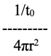(1)
These field lines must get close enough to the second singleton point to increase the elementary time unit slightly so that the singleton point can travel a slightly larger distance in the same amount of time as measured by a stationary observer, therefore creating acceleration, since its velocity as far as the stationary observer is concerned in order to create a force. In a quantized space “close enough” means exactly x0 and the area near the singleton point that these field lines must pass through is (x0)2. The force from these electric lines on the second electron is the number of these lines that pass through this area. The equation for this force is: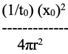(2)
The equation for the force between two electrons was experimentally determined by Coulomb around 1800 and it has been used ever since. It is: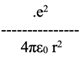(3)
Where e is the elementary charge of the electron which is squared since the two electrons involved contribute charge and ε0 is called the permittivity of free space and is a constant of free space.
Since (the elementary spacial distance) equals (the speed of light) times (the elementary time unit) or x0 = ct0 is the relationship between space and time quantization. With this relationship, we have: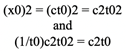(4)
Now if both equations for the electric force were set equal to each other it would look like: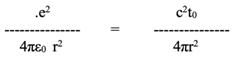(5)
And, if the two equations are equal, then the following relationship must hold: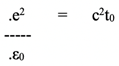(6)
The speed of light is related to space and time using the following equation involving the constants of free space μ0 (the permeability of free space) and ε0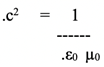(7)
Now, from the previous equation (7), substituting for c, we have: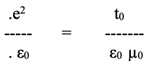(8)
Then, solving for the electric charge e, we have: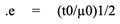(9)
where the ½ power means square root and which mathematically relates the elementary unit of charge, e, to the elementary unit of time, t0.
From the measured value of e = 1.602177 x 10-19 C where C is the charge unit Coulomb.
The calculated value of t0 is 3.2343 x 10-44 seconds or (3/5) tp where tp is the Planck time.
For continuous time, t0 = 0, so e = 0 and there can be no electron.
The elementary time unit, t0, that was found from the calculation was exactly three-fifths times the Planck time (considered by most to be the smallest quantity of time).
3/5 is not an arbitrary fraction. 3/5 is part of the 3-4-5 triangle which is the only triangle that has integral (whole number) steps on all three sides which goes along perfectly with the quantization of time. If time does progress in integral steps, then time would have to travel along the path of one of the sides of this triangle.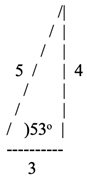(10)
3 is our side of time and our time progresses at a rate (3/5) times the Planck time which represents the (5/5) side of the triangle. The two sides (5/5) and (4/5) may be considered dark matter since their different time unit would mean that they cannot interact with our matter. (more information about this theory can be found online at sciencepub.net Academic Arena Vol 5 #9 2013 5(9) titled “The Derivation of Dirac’s Equation” by George Gerhab) My.

### 5. Energy of the Micro-Electric Field Lines

Each tiny electric field line that is created by the singleton point has a small amount of energy associated with it. The two points of a virtual pair become “unsynchronized” because the point next to it, which will suddenly become the singleton point, lasts longer (once as virtual and once as real) and can transfer an impulse to the virtual pair in front. The point cannot disappear with the point next to it because the new singleton point would move 2x0 in a time t0 meaning it would be traveling twice the speed of light which is not allowed.
This energy takes the form of the “unsynchronization” of the pair’s time vectors and the virtual can no longer disappear and becomes a micro-electric field line. Consider the following diagram: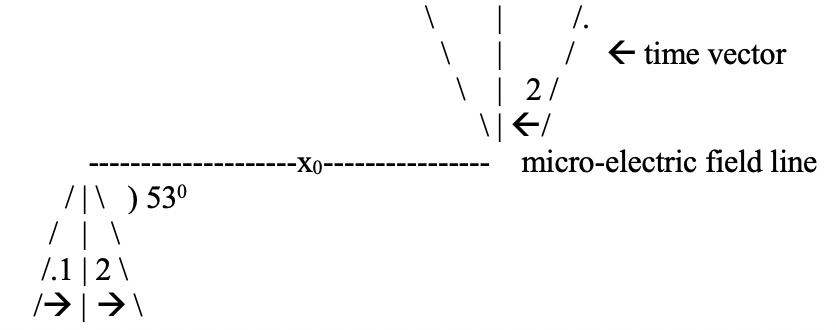Figure 5
The bottom time vector starts at 53° and goes backward in time, initially, which would make it a positron, since it is 180° out of phase with the electron. If the pair is knocked out of synch, then step 1 on the bottom would not correspond to step 2 on top and these points would not be able to disappear, but rather travel outward, possibly giving its impulse to another charged particle.
The impulse given to this pair would have to depend upon the time, t0, which the time that the impulse is given, and the strength of the force giving it this impulse, namely (4πε0)-1. Since there are two points involved, these would have to be squared: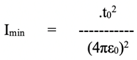(11)
The equation for the Plank time is: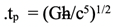(12)
While for our elementary unit of time, we have: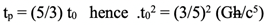(13)
Inserting this into the equation for Imin yields: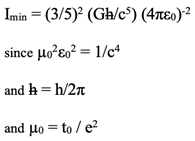(14)
Where G = the Gravitational Constant =
6.672 x 10-11 N-m2/kg2
.e = elementary unit of charge = 1.602 x 10-19 C
.c = speed of light = 3 x 108 m/s
.μ0 = 4π x 10-7 N(s/C)2 and taking the 4π out into Imin yields: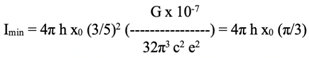(15)
The calculation: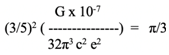(16)
has a great deal of implication, π/3 is the exact number of radians in 60° which means that in Imin this would be (π/3)x0 which would form an arc between points, keeping the transfer of the impulse, Imin, exactly the distance x0 away from all other points in space-time. This seems to mean that 5 very well-known physical constants are “conspiring” to make sure that everything in space is exactly x0 away from each other and that the only angle in space allowable is 600.
The Actual calculation is 1.04798 while π/3 = 1.047194, which I think is pretty close. Hence: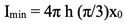(17)
Since this calculation is so accurate and so meaningful, it cannot be coincidental. As such, it lends validity to the elementary time unit since (3/5)2 is part of the calculation as well as the equation t0 = e2μ0, which also was used in this calculation.
The units of this is m2/C4 which, actually, makes sense since two virtual pairs (having time which is associated with each point consequently charge) hence an area (m2) can indeed be associated with the charge unit to the fourth power.

### 6. The Magnetic Field and Special Relativity

In my theory the micro-electric field line must not be seen as travelling faster than light from any inertial reference frame.
If an inertial reference frame is traveling at a speed, v, in the opposite direction that the micro-line is, this would look like the micro-line could be traveling faster than light and endangering the integrity of quantized space time.
Instead of the micro-electric line being seen as traveling faster than light, I propose that another field arises, perpendicular to the E-line and dependent on the velocity, v, in order to preserve the integrity of space-time. This field is the well-known magnetic field.
Graphically in figure 6: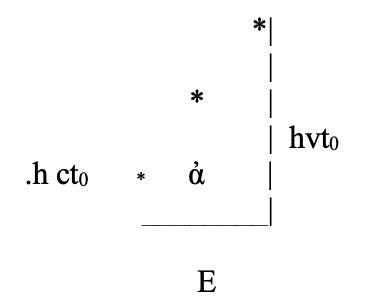Figure 6
Where sinἀ = (hvt0)/(hct0) = v/c
So that the left side of the law of tangents becomes: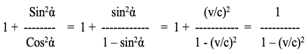(18)
In order to get the real velocity from the magnetic field velocity, a rotation of 90° is needed. Hence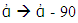and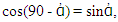so that the right side of the law of tangents becomes sec2 =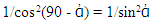= (p/mv)2, where mv is what the momentum would be in a continuous space-time and infinite speed limit, and p is the actual momentum as seen by an inertial reference frame that sees the particle traveling at speed v. Hence, the law of tangents then becomes: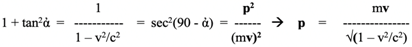(19)
The magnetic field, B, is related to the electric field, E, by B = E x (v/c) and the magnetic force is related to the electric force by: Fmag = F (v1 x v2)/c2. Using the equation for the electric derived earlier: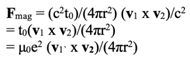(20)
Since t0 = μ0 e2

### 7. The Gravitational Field This is How My Theory Predicts How the Gravitational Field Comes about from the Micro-Field Lines

As stated earlier, when a singleton point skips a distance x0 it creates a micro-field line that travels outward at the speed of light and creates the electric force. As also stated, these micro-lines sweep over a distance of (π/3)x0 (see eq 16) to maintain the elementary distance from space-time points.
The volume created by a pair (one from a + charge and one from a – charge) would be (π/3)2x02(2πx0) since there would be two π/3 sides and one circumference. These larger elementary volumes would be occurring on a temporary basis at a frequency dependent on the gravitating mass, M, and the distance, r, from the mass.
The number of micro-field lines emitted by a massive object, M, and occurring at a distance, r, is:
A = # field lines at: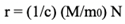(21)
N = 1/t0 = #lines emitted by one charged particle, t0 is the elementary unit of time, m0 is the elementary unit of mass (nucleon + electron) division by c is because we want the number at the distance r only.
B = # elementary volumes at: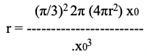(22)
4πr2x0 = volume in a thin sphere, the rest is the increase due to the micro-line pairs of the electric field.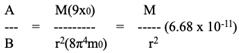(23)
The gravitational force is due to the frequency of the increases and decreases of the elementary volume of space due to the electric micro-field lines.

### 8. The Impulse and Wave Equations

In my theory, the energy given by this electric micro-impulse, Imin, depends on the uncertainty of the singleton point, which is traveling at the speed of light within a distance of ∆x, and as such, its position can never be exactly determined. The energy of these micro- impulses is determined by how close, ∆x, they are to each other and the singleton point. The total energy of the f (one field is multiplied by how many movements the singleton point has per second, N = 1/t0. Hence, the total uncertainty of the energy of the field is: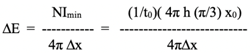(24)
Since x0/t0 = c this becomes: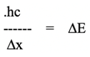(25)
Since ∆x = c∆t and ∆E = ∆p c this becomes: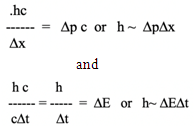(26)
If ∆x can be considered a wavelength, λ, and 1/∆ = f, a frequency, then these equations would become h/λ = p and h f = E. Also consider the following: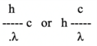(27)
They seem to be the same, yet h/λ does not infer that the wave is traveling at the speed of light, whereas c/λ does. E = pc is the energy of the field, which is traveling less than the speed of light, while E = hf is the energy of the singleton point which is traveling at the speed of light. It seems that there are two waves simultaneously being carried out in the electron. A singleton point wave (governed by Dirac’s equation) and a field wave consisting of all the micro-fields lines that have been saved and became part of the particle’s field (governed by the Kline-Gordon equation).
The Wave
The time motion of the time vector of the singleton point is: according to my theory, the quantum wave is caused the time vector of the singleton point.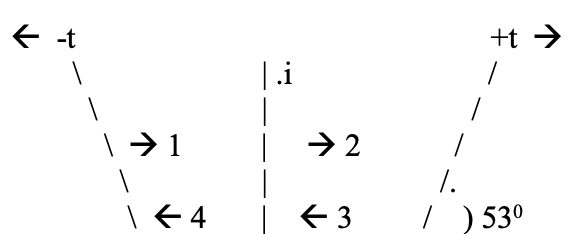Figure 7
The electron starts at negative t0cos53° or –(3/5)t0 and initially moves forward in time for two time clicks to +(3/5)t0, then it moves backward in time (3 & 4) for two time clicks, then it moves back and forth, indefinitely. Each time motion requires a separate time function: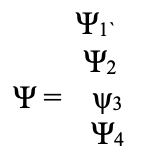Figure 8
Each of these functions can have either + or – ½ spin since the only angle allowed by quantize space is 60° and the cos 60° = ½, the exact spin of the electron.
These functions can change spin and order as such: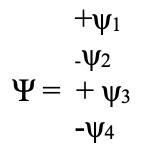Figure 9
These functions can change places with the positive time movements.
The possibilities for the exchanges in the four columns can be seen in figure 10: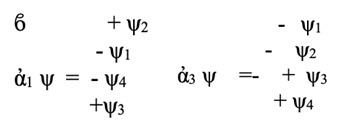Figure 10
The 2nd matrix has imaginary numbers in it, which means that the imaginary part of the wave function plays a real role in the solution of the Dirac function. Since sine(53) = cos(37), it seems to me that 37° is the phase factor for a part of dark energy, since it’s the only other time phase factor that is possible in the 3-4-5 triangle which represents the only integral time movements that are possible if time moves in integral steps.
The other Dirac matrix,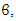has the reverse time functions reversed to the top two functions in the function column. This is more like a positron function which starts at +t0 and moves backward in time, 180° out of phase with the electron.
With these Dirac matrixes, the Hamiltonian becomes: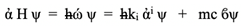(28)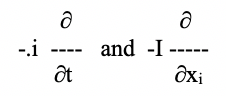Figure 11
are the energy and momentum operators hence: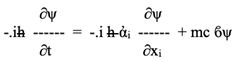(29)
This is Dirac’s equation, mostly electron with dark matter and positron mixed in.

### 9. The Muon and the Family of Particles, My Idea of More Complex Points

A micro-field line is a + and a – point exchanging time rapidly and causing space-time to oscillate between + and – but cannot disappear because they’ve been given a slight “tug” of Imin. When two such sets of microline points come close enough (within a distance X0 which would occur in high energy collisions) two points, one from each pair, can exchange time and disappear, leaving the other two points separated by 2X0 and are free and can become enveloped into an electron’s field, creating a three-point muon. Two of the points eventually get close enough to exchange time which neutralizes the points and become neutrinos. The electron is left over.
The anomalies of the muon’s magnetic moment and its anomaly of increasing the size of the proton can be explained by this explanation of the muon. Three points rather than one would have a different magnetic moment than the electron. While orbiting a proton, the two negative points of the three would be closer to the proton, enlarging it more than the electron would.
Anti-Gravity a proposal
Just like the magnetic field appears in order to keep the electric micro-lines from appearing to travel faster than light speed (which would affect the elementary units of time and space) a magnetic like field appears to prevent the motion of the gravitational field density from traveling faster than light speed as well.
Gravitational objects traveling at some speed measured by an inertial reference frame must have a field perpendicular to the normal gravitational field. If two such gravitational objects are traveling in the same direction, according to a third inertial frame, they would seem to repel each other similar to the way currents in the same direction do. Only extreme speeds (distant galaxies traveling away from us at close to light speed.) would cause this repulsion.
Distant galaxies traveling close to light speed would have an enormous mass-energy (E = mc2/√(1-v2/c2)) and could repel all similar galaxies which would expand the space between them and drag the rest of the universe with it.
When the universe began, matter was blown straight out, very near the speed of light, and the perpendicular repulsion of gravity would expand the universe suddenly.
In galaxies, one arm of the spiral is traveling in the opposite direction as the spiral arm on the opposite side of the center. In magnetism, two currents traveling in opposite direction attract. The two spiral arms would act in the same way, and give an addition attraction in the galaxies, not to mention the super-massive black holes that are found in each and every one of them.
Electro-magnetic waves are formed by two forces that are perpendicular to each other caused by the fact that no force field can travel faster than light (inherent in the basic fabric of all space-time). Gravitational waves would indicate that there exists an anti-gravity force perpendicular to the normal gravity field, resulting from the fact that the gravity field, like the electric field, cannot travel faster than the basic speed within the fabric of all space-time.
In the beginning, when the universe blew up, matter was traveling outward at near this speed, and repelled each other and expanded the universe suddenly. The universe has been expanding ever since.
The recent measurement of gravity waves does now seem to verify this, and it also seems to show that these forces are one in the same.
Big Bangs, purely hypothetical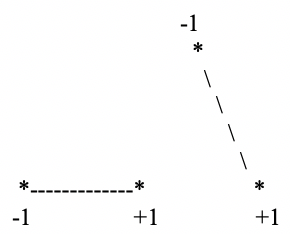Figure 12
Two virtual pairs blink as shown, but instead of the pairs blinking normally, the above -1 point tries to share time with two +1 points at the same time. Since only one elementary time unit is allowed during each integral step of time, this would be impossible, but in an extremely rare case only a fraction of the time unit would, because of entanglement, be exchanged and create partial charges, -1/3, +2/3. They are held together by entanglement gone wrong.
Since these points become real, and last a long time, they polarize space-time and the once highly unlikely event now occurs every t0 seconds and 1/t0 number of electrons and protons occur every second. When the points become real, they create extra space which causes the particles to fly out.
The other two types of matter (time phase angle 37° and 0°) have a larger elementary time unit, (4/5)tp and tp, they have a better chance at creation then our matter does, which is 1 chance in 4. Also, since the elementary field unit (e = √(t00)) is larger for dark matter, it would have more mass. A ratio of 5 to 1, dark over our matter, is not inconsistent with my theory.
Black Holes
They are the saturation of matter and space-time?.

### 10. Conclusions

My unification theory has one particle and one force which, I believe, my math confirms.

### References

  Gerhab, George, “The Derivation of Dirac’s Equation”, Academic Arena, 2013, 5(9), sciencepub.net/academia 2013, 5(9).  W.N. Cottingham and D.A. Greenwood, “An Introduction to the Standard Model of Particle Physics” 2007 Cambridge University Press ISBN-13 978-0-521-85249-4.  David R. Lide, Editor in Chief, “The Handbook of Chemistry and Physics” 1993-1994 edition.